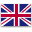Shenmao Capacitors specialized in aluminum electrolytic capacitors from 1970

ShIP toEnglish

# Is LC resonant circuit application too difficult? In fact, this step is very important

by：Shenmao     2021-07-05
According to the different connection modes of the inductor L and the capacitor C in the circuit, there are two basic LC resonant circuits: LC parallel resonant circuit and LC series resonant circuit. In amplifier circuits and other forms of signal processing circuits, a large number of LC parallel resonant circuits and LC series resonant circuits are used. The following figure is the application description of the LC resonant circuit: LC parallel and series resonant circuits have many changes in the application, which is a difficult point in circuit analysis. 1. LC free resonant circuit The figure below shows the LC free resonant circuit. In the circuit, L1 is an inductor, C1 is a capacitor, and L1 and C1 form a parallel circuit. As shown in the figure below, the basic resonance process of the LC resonant circuit is: suppose that there is electric energy in the capacitor C1 at the beginning, and then the electric energy of C1 discharges to L1. This process is the process of converting the electric energy in C1 into magnetic energy in the coil L1. When the capacitor C1 discharges, all the energy is stored in the coil L1 in the form of magnetic energy. As shown in the figure below, after C1 is discharged, the magnetic energy in the coil L1 charges C1 by the self-induced electromotive force at both ends of the coil to charge C1. This charging process is the process of converting the magnetic energy in the coil L1 into electric energy in the capacitor C1. After the capacitor C1 is charged, the voltage across the capacitor discharges the coil again, and another cycle of oscillation and energy conversion begins. 2. LC parallel resonant circuit The figure below shows the LC parallel resonant circuit. L1 and C1 in the circuit constitute LC Parallel resonance circuit, R1 is the DC resistance of the coil L1, Is is the AC signal source, which is a constant current source. The so-called constant current source is a power source whose output current does not change with the size of the load. In order to facilitate the discussion of the LC parallel circuit, the coil resistance R1 can be ignored, and the simplified circuit is shown in the figure below. The impedance of the LC parallel resonant circuit can be equivalent to a resistor, which is a special resistor, and its resistance value changes with the frequency. This equivalence can facilitate the understanding of the working principle of the circuit. An important characteristic of the LC parallel circuit: the impedance of the circuit reaches its maximum when parallel resonance. After the input signal frequency is higher than the resonant frequency, the LC parallel resonant circuit is equivalent to a capacitor. ①Impedance characteristic curve when the input signal frequency is equal to the resonance frequency: ②Impedance characteristic curve when the input signal frequency is higher than the resonance frequency: ③Impedance characteristic curve when the input signal frequency is lower than the resonance frequency: ④LC parallel resonance network reactance characteristic curve: ⑤LC parallel resonance circuit The frequency characteristic curve of the circuit: 3. LC series resonant circuit LC series resonant circuit is another kind of resonant circuit in LC resonant circuit. The figure below shows the LC series resonant circuit. In the circuit, R1 is the DC resistance of the coil L1, and is also the damping circuit of this LC series resonant circuit. The resistor is an energy dissipating element, which consumes the energy of the resonant signal here. L1 and C1 are connected in series and then connected in parallel with the signal source, where the signal source is a constant voltage source. In the LC series resonant circuit, the smaller the resistance of the resistor R1, the smaller the energy consumption of the resonant signal, the better the quality of the resonant circuit, and the higher the Q value of the circuit. When the inductance L1 in the circuit is larger, the magnetic energy stored is more. When the circuit loss is constant, the quality of the resonant circuit is better, and the Q value of the circuit is higher. In the circuit, there is no energy conversion between the signal source and the LC series resonant circuit, but there is a conversion between electrical energy and magnetic energy between the capacitor C1 and the inductor L1, and the external input signal only supplements the loss due to the electrical energy consumed by the resistor R1 Signal energy.
Shenzhen Shen MaoXin Electronics Co., Ltd. is fully committed to supplying high quality products and services.
Shenzhen Shen MaoXin Electronics Co., Ltd. builds value for our investors through the strength of our customers’ satisfaction and by consistently producing superior operating results.
When it comes to electrolytic capacitor electrolytic capacitor suppliers, Shenzhen Shen MaoXin Electronics Co., Ltd. is the name to reckon with. Not only are they best, they are the most experienced as well and provide wide range of services as well as products at affordable prices. Find out more information on Shenmao Capacitors.
Among improvements to electrolytic capacitor, nearly half of consumers considered quality and service as the most important change a business could make in its supply chain.
Our company is professional in selling electrolytic capacitor as well as providing a series of relevant services.
Custom message
Chat Online 编辑模式下无法使用